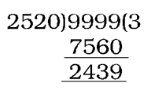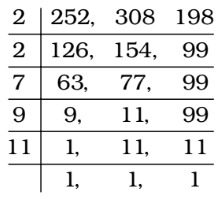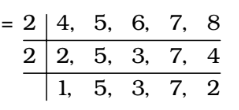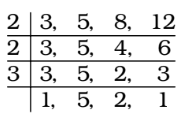## LCM and HCF

#### LCM and HCF

1. The least perfect square, which is divisible by each of 21, 36 and 66 is

1. LCM of 21, 36 and 66
∴ LCM = 3 × 2 × 7 × 6 × 11
⇒ LCM = 3 × 3 × 2 × 2 × 7 × 11
∴ Required number = 32 × 22 × 72 × 112

##### Correct Option: C

LCM of 21, 36 and 66
∴ LCM = 3 × 2 × 7 × 6 × 11
⇒ LCM = 3 × 3 × 2 × 2 × 7 × 11
∴ Required number = 32 × 22 × 72 × 112
Required number = 213444

1. Find the largest number of four digits such that on dividing by 15, 18, 21 and 24 the remainders are 11, 14, 17 and 20 respectively.

1. As per the given details in question , we have
15 = 3 × 5
18 = 32 × 2
21 = 3 × 7
24 = 23 × 3
LCM of 15 , 18 , 21 and 24 = 8 × 9 × 5 × 7 = 2520
The largest number of four digits = 9999##### Correct Option: B

As per the given details in question , we have
15 = 3 × 5
18 = 32 × 2
21 = 3 × 7
24 = 23 × 3
LCM of 15 , 18 , 21 and 24 = 8 × 9 × 5 × 7 = 2520
The largest number of four digits = 9999Required number = 9999 – 2439 – 4 = 7556
(Because 15 – 11 = 4
18 – 14 = 4
21 – 17 = 4
24 – 20 = 4)

1. A, B, C start running at the same time and at the same point in the same direction in a circular stadium. A completes a round in 252 seconds, B in 308 seconds and C in 198 seconds. After what time will they meet again at the starting point ?

1. Required time = LCM of 252, 308 and 198 seconds∴ LCM = 2 × 2 × 7 × 9 × 11

##### Correct Option: D

Required time = LCM of 252, 308 and 198 seconds∴ LCM = 2 × 2 × 7 × 9 × 11
Required time = 2772 seconds
Required time = 46 minutes 12 seconds

1. The least multiple of 13, which on dividing by 4, 5, 6, 7 and 8 leaves remainder 2 in each case is:

1. According to question ,
LCM of 4, 5, 6, 7 and 8LCM of 4, 5, 6, 7 and 8 = 2 × 2 × 2 × 3 × 5 × 7 = 840.
Let required number be 840K + 2 which is multiple of 13.
Least value of K for which ( 840K + 2 ) is divisible by 13 is K = 3

##### Correct Option: C

According to question ,
LCM of 4, 5, 6, 7 and 8LCM of 4, 5, 6, 7 and 8 = 2 × 2 × 2 × 3 × 5 × 7 = 840.
Let required number be 840K + 2 which is multiple of 13.
Least value of K for which ( 840K + 2 ) is divisible by 13 is K = 3
∴ Required number = 840 × 3 + 2
Required number = 2520 + 2 = 2522

1. Find the greatest number of five digits which when divided by 3, 5, 8, 12 have 2 as remainder :

1. Here , Remainder ( r ) = 2
As we know that When a number is divided by a, b , c or d leaving same remainder ‘r’ in each case then that number must be k + r where k is LCM of a, b , c and d.
The greatest number of five digits is 99999.
LCM of 3, 5, 8 and 12∴ LCM = 2 × 2 × 3 × 5 × 2 = 120
After dividing 99999 by 120, we get 39 as remainder
99999 – 39 = 99960 = ( 833 × 120 )

##### Correct Option: D

Here , Remainder ( r ) = 2
As we know that When a number is divided by a, b , c or d leaving same remainder ‘r’ in each case then that number must be k + r where k is LCM of a, b , c and d.
The greatest number of five digits is 99999.
LCM of 3, 5, 8 and 12∴ LCM = 2 × 2 × 3 × 5 × 2 = 120
After dividing 99999 by 120, we get 39 as remainder
99999 – 39 = 99960 = ( 833 × 120 )
99960 is the greatest five digit number divisible by the given divisors.
In order to get 2 as remainder in each case we will simply add 2 to 99960.
∴ Greatest number = 99960 + 2 = 99962